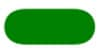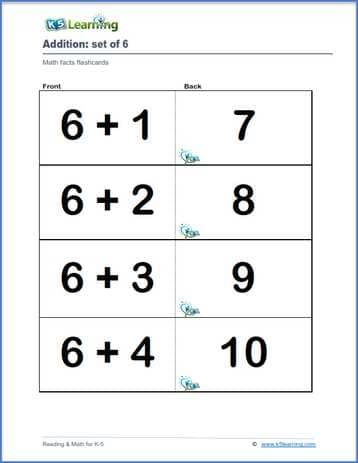# Math Flashcards

## Printable math flashcards

These flashcards help students learn their addition, subtraction, multiplication and division math facts.  Cut out each math fact as a separate card. Then fold each card in half with the question on the front and the answer on the back of the card.

 Addition flashcards: set of 0 to 12 addition math facts flashcardsSubtraction flashcards: set of 0 to 9 subtraction math facts flashcardsMultiplication flashcards: set of 0 to 12 multiplication math facts flashcardsDivision flashcards: set of 0 to 12 division math facts flashcardsSample math facts flashcards

What is K5?

K5 Learning offers free worksheets, flashcards and inexpensive workbooks for kids in kindergarten to grade 5. Become a member to access additional content and skip ads.